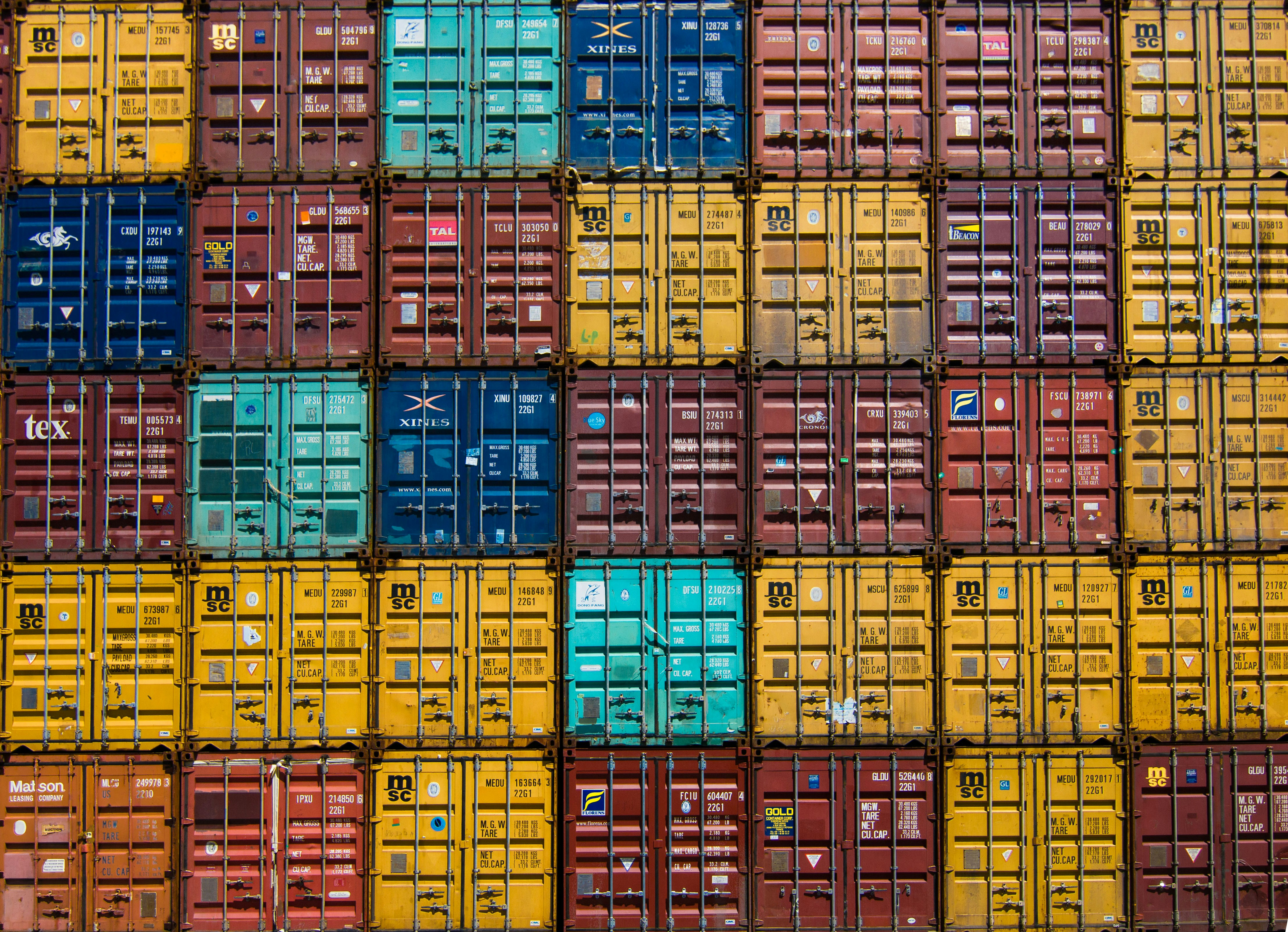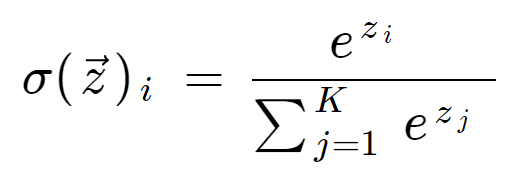Akhil Chaudhary
NIT Warangal graduate, Software Engineer at Wipro.

# Softmax FunctionThe softmax function is a special case of the logistic function, where it is applied to a multi-class problem. It takes a vector of K real values and converts it into a vector of K real values that sum up to 1. In other words, the input gets normalized to a probability distribution that is proportional to the exponentials of the input numbers.

The softmax function is often called multi-class logistic regression as it is a generalization of the latter applied to multiple classes. Its formula is also very similar to the sigmoid function used in logistic regression.

Multi-layer neural networks also often use softmax as the penultimate layer in the network. Hidden layer outputs are unscaled and therefore difficult to analyze and interpret. The softmax function converts these values into a probability distribution. Probabilistic outputs allow for easy classification of inputs. Larger inputs correspond to larger probabilities.

# FORMULAfor input vector z having K number of classes.

# CODE

import numpy as np

def softmax_numpy(scores):

return np.exp(scores)/sum(np.exp(scores), axis=0)

# SOFTARGMAX

The softmax function is sometimes explicitly referred to as the softargmax function. The argmax function takes a vector and converts every value into zero, except the maximum value, where it returns 1.

The softmax function is differentiable in training and allows to optimize the cost function. However, we sometimes need the model to predict a single value for inference. In such cases, argmax is most useful. The value it returns corresponds to the class for which the probability is maximum.

CONCLUSION

In recent years, with the growing popularity of neural networks, the softmax function has become well known due to its excellent utility in generating probabilistic distributions. This has led to its widespread use in multi-class classification systems and neural networks training.